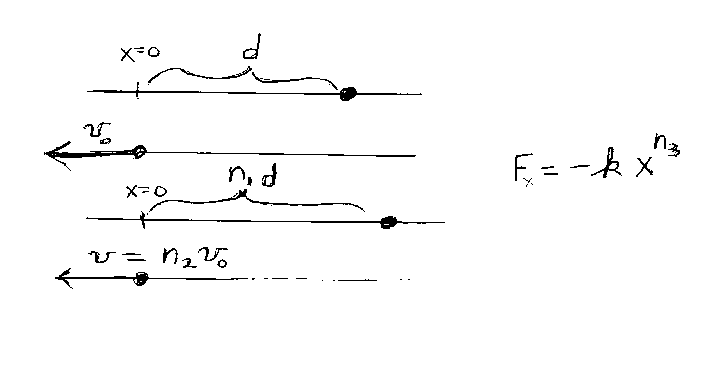Problem C16: An object of mass m, which is confined to move on the "x-axis", is subject to a resoring force F. You know that the magnitude of F is proportional to xn3, where x is the position on the x-axis. You want to determine the exponent n3, so you carry out two experiments. You bring the object out to the position x=d and release it from rest, it returns to the origin (x=0) where you measure it's speed to be v0. Now you bring the object out to the position x=n1d and release it from rest. You measure it's speed v as it passes though the origin at x=0 and obtain v=n2v0. With this information, determine the exponent n3? Note that n1, n2 and n3 are unitless.n1 = n2 = Input n3:
If you are currently in my class, you can record your grade by entering your name and student ID number (without the leading zeros) below and clicking on "record grade".
 First Name = Last Name = ID = Problem: